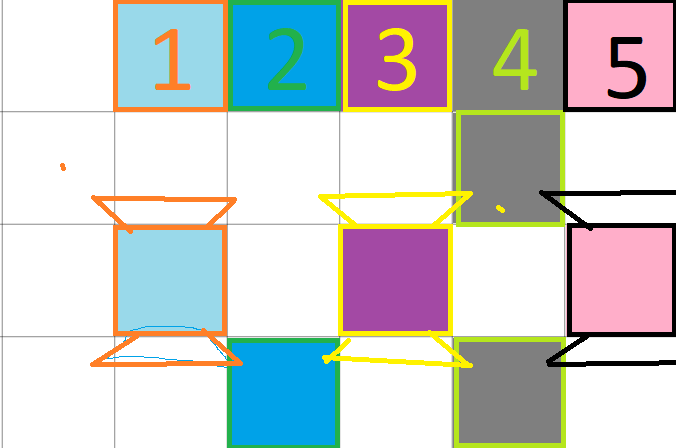# how to describe diagonal clones of centre object?

i’m spawning same object but want to delete diagonal clones. should i give variable for every random spawning same object? by the way they are not colliding like chessboard.

1// whether it is top-left,top-right etc one,its distance between center clone will be same .i use compare 2 expressions like
f(x)kutu.Distance(kutu)=10*sqrt(2) but cant delete in action.how can i describe non-center clones?

2//with “distance with 2 objects”,it stands for “box distance to box is below sqrt(pow2(x1-x2)+pow2(y1-y2))” .in this condition because of distance never goes below in diagonal ones ,it deletes side by side ones

3//compared angle between objects : box is rotated towards box ( 44.5) ; delete object.but it deletes some of them .

https://app.box.com/s/djjz57t1m9k5a0c52hperzzq8rrddiyb

The 1 and 3 methods should work, the problem is the way GD handles instances, you can’t just do Box.Distance(Box) because GD doesn’t know which Box respect to which Box check the distance, as the Box instances taken into account for the event stand in a list the result is unpredictable I think, maybe it will check every Box object distance to every Box except iself, not sure…

What you have to do is add a “token”, a placeholder for the first Box: Add a new object, for example a sprite (if you’ll check position any kind of object can do the trick) and set it position = position of the central Box.
Now instead deleting the Boxes at X distance from the central Box, you have to delete the Boxes at X distance from the Placeholder. The Boxes to delete and the Placeholder to check distances are different objects so GD will have no problemSome things to note:

• Distance functions measure from the objects center, so you have to place the Placeholder in a way that the Placeholder Centre and Box Centre points overlap (for example put the Origin point of the Placeholder at the Centre point and create the Placeholder at the Centre point of the Box).
• If you use the distance method you should use a little tolerance factor too (a range), because float precision. You will have to add a condition per possible distance to the central box (diagonal range 1 (first radius), diagonal range 2 (second radius), etc.).
• The best coding way (I think) to do what you want, the natural one, is to use angles, I think there is no condition to check angles between objects (there is one to check angle of movement between objects I think, sorry, no GD in this PC, checking the sources), so you’ll have to test the distances manually, and add a little tolerance angle too. If you know some trigonometry, the dot/cross products can make the things easier* Did you try to make a big cross sprite with a cross collision mask, and delete the Boxes colliding with the cross? That would be super easy!if i do that the box next to it also will destroy, i just wanna destroy diagonal ones and also it makes colliding with other objects different.even i put a placeholder moves with box and has larger mask , it ll still delete right and left ones.

but i added a placeholder and replaced it to the origin of box with same speed so they are in same position always.

if i cant delete diagonals in GD, i must improve randomizations of spawning.

Check this:
DeleteDiagonal.zip (6.18 KB)
There are 3 scenes:

• One with a fixed mask, the mask was made specifically to delete the diagonals in a distance of 2 boxes, it’s super easy but if you want to delete more objects, for example a distance of 3, you have to add the collision masks in the object manually.
• One with a dynamic mask, the mask is only a placeholder to check collisions, the code generates the masks in all the diagonals in a distance given, then just delete every box colliding with a mask.
• The last one is pure logic, it calculates positions of every possible diagonal box, in the given distance, then check if a box is close to the calculated position.

As you can see, there are two factors to balance: complexity and functionalitythaank you so much
i did a fixed mask also like thisbut yours is more handy. and i got the logic of calculations but cant get this part in last example;

It checks if the real Box position is close enough to the calculated position, the conditions represent a little square from the calculated position, 5 pixels to the left, right, up and down, and check if the Box position is inside this little square